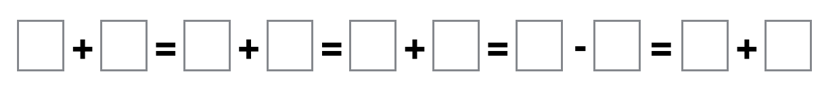Home > Grade 1 > Equality

# Equality

Directions: Place any digit, 0 through 9, in the boxes below to make a true statement.  Each digit can only be used once.### Hint

How can we pair up the numbers so that all the pairs are equivalent?

Each pair should add up to 9, for example 4 + 5 = 1 + 8…and so on

Source: Graham Fletcher

## Adding with Parenthesis

Directions: Using the digits 1 to 9, at most one time each, make a true …

### One comment

1.This is an example of running equal signs. There can only be one equal sign in an equation.